用C++做資料分析 | CERN ROOT 教學 - 函數與函數擬合

函數

ROOT的TF1可以用來表示任意的一元函數。他的自變數的名稱永遠為x。而且你可以在裡面使用任意的C/C++函數(包括ROOT自帶的TMath函數庫)。

root  f1 = new TF1("f1", "sin(x)/x", -10, 10);
root  f1->Draw();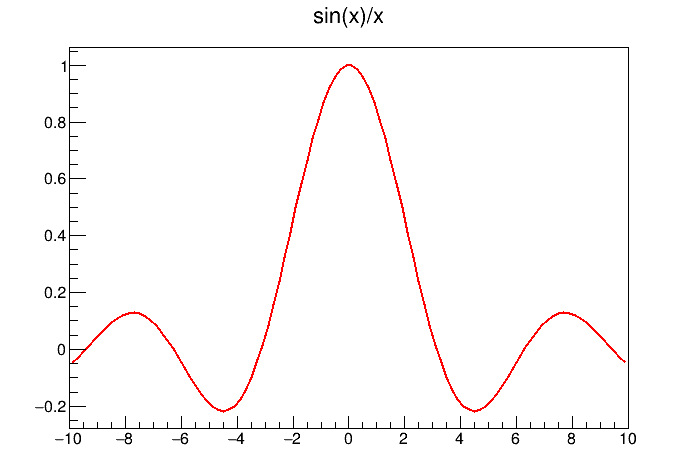sin(x)/x

root  my_sinc = function([](double x){ return sin(x)/x; });
root  f1 = new TF1("f1", "my_sinc(x)", -10, 10);
root  f1->Draw();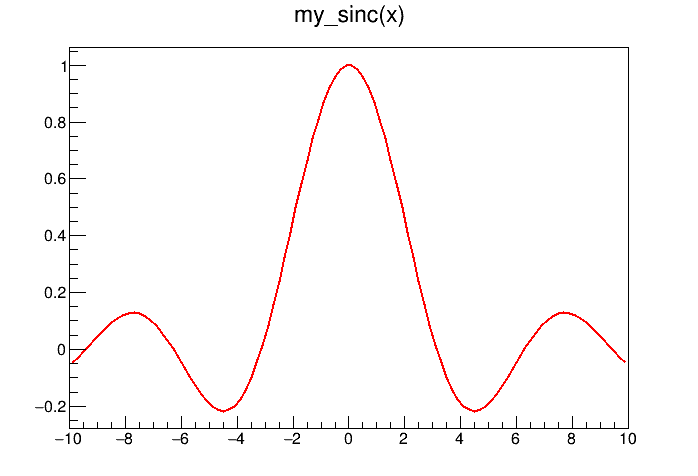sin(x)/x

root  f1 = new TF1("f1", "*x+", -10, 10);
root  f1->SetParameters(-1, -5);
root  f1->Draw();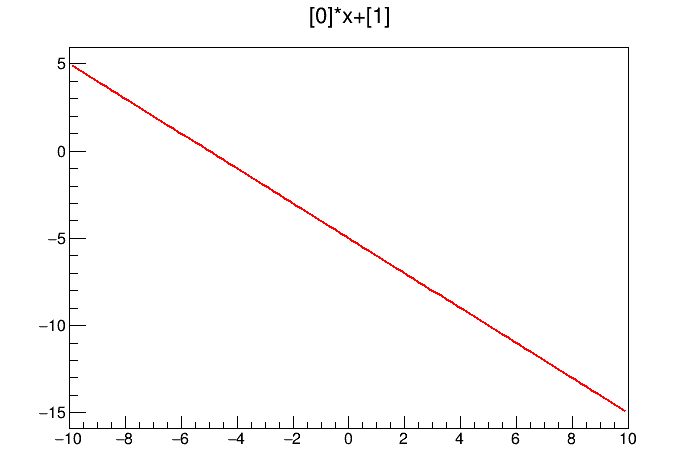y = ax+b，a = -1, b = -5

root  f1 = new TF1("f1", "exp(x+[a])", -10, 10);
root  f1->SetParameter("a", 2);
root  f1->Draw();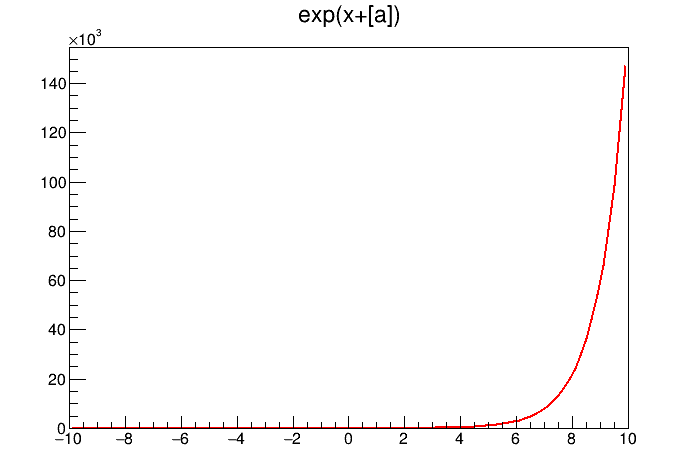exp(x+a)

root  f1->GetParameter("a")
(double) 2.0000000

函數擬合

root  h1 = new TH1F("h1", "histogram", 60, -3, 3);
root  h1->FillRandom("gaus", 100000);
root  h1->Draw();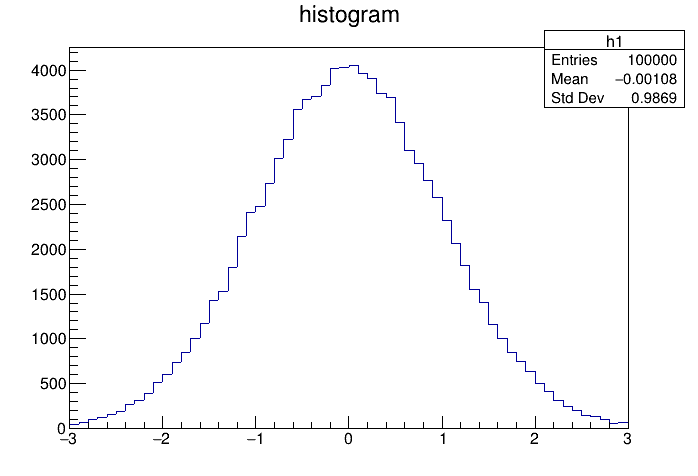root  h1->Fit("gaus");
FCN=58.2284 FROM MIGRAD    STATUS=CONVERGED      62 CALLS          63 TOTAL
EDM=8.58021e-13    STRATEGY= 1      ERROR MATRIX ACCURATE
EXT PARAMETER                                   STEP         FIRST
NO.   NAME      VALUE            ERROR          SIZE      DERIVATIVE
1  Constant     4.00036e+03   1.57642e+01   4.75510e-02  -9.85101e-09
2  Mean        -1.17823e-03   3.20398e-03   1.20393e-05  -2.62278e-05
3  Sigma        9.99354e-01   2.41238e-03   2.44449e-06  -1.71898e-03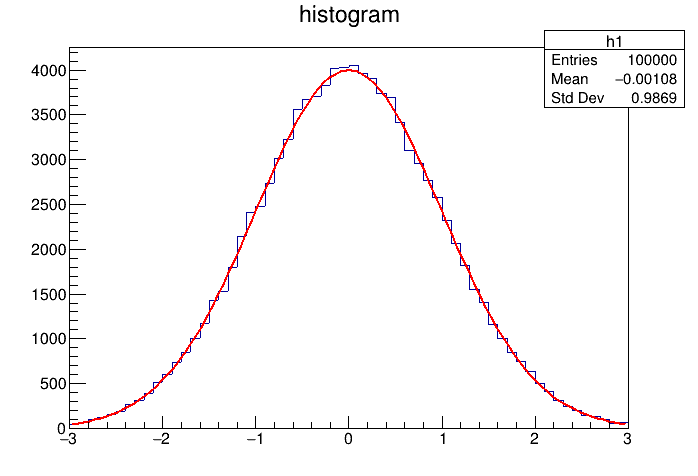root  f1 = h1->GetFunction("gaus");
root  f1->GetParameter("Constant")
(double) 4000.3641
root  f1->GetParameter("Sigma")
(double) 0.99935417

擬合自定義函數

root  f2 = new TF1("f2", "[scale]*sin(x)/x+[bias]");
root  h1->Fit(f2);
FCN=14310 FROM MIGRAD    STATUS=CONVERGED      30 CALLS          31 TOTAL
EDM=7.17638e-19    STRATEGY= 1      ERROR MATRIX ACCURATE
EXT PARAMETER                                   STEP         FIRST
NO.   NAME      VALUE            ERROR          SIZE      DERIVATIVE
1  bias        -3.32813e+02   3.54589e+00   1.38923e-01   2.29136e-10
2  scale        2.85750e+03   1.11800e+01   4.38015e-01  -4.15280e-11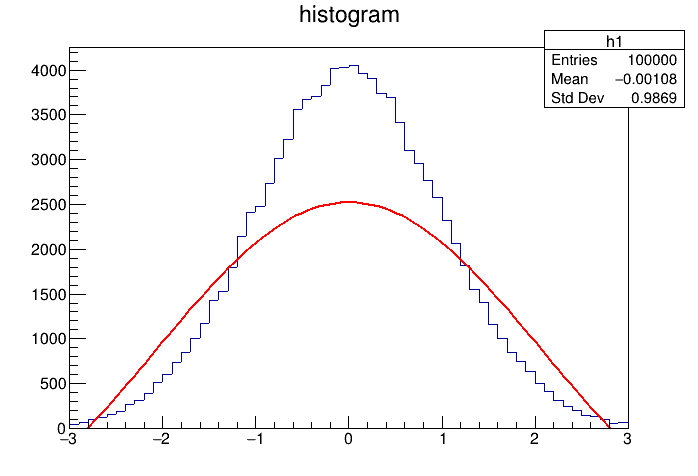#CERN, ROOT